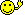CodeGuru Home VC++ / MFC / C++ .NET / C# Visual Basic VB Forums Developer.com

1.Junior MemberJoin Date
Oct 2006
Posts
1

##Do While and Adding

I need to write a code using the Do while statement. I have a input box that comes up and you put in a number and then the code needs to be so that it adds all the numbers that come before the one enteredand that one. So if you put in 10 it'll ad 1 + 2 +3 +4, ect.. all the to 10 and then displays the total in a message box. My problem is I'm running off of three hours sleep and I need this done tonight. I cant figure out for nothing what equation i need to use. PLEASE HELP ME!!!!Reply With Quote

2. ## Re: Do While and Adding

the logic goes something like this.
Code:
```y=0 : z=0
Do while y<=x
z=z+y
y=y+1
if y>=x then exit do
loop
msgbox "Print Total:"+ str\$(z)```
Last edited by HanneSThEGreaT; October 28th, 2006 at 02:48 AM. Reason: Code TagsReply With Quote

3. ## Re: Do While and Adding

Hello & welcome to the Forums SkyelarJoe!So, if I understand you correctly, this is what you want to do :
You want to enter a number into an InputBox, example 10
Then you want to add all the numbers up to 10, like :
1 + 2 + 3 + 4 + 5 + 6 + 7 + 8 + 9 + 10

That will amount to 55
doing the same with the inputted number 20 will end up being 210

Then this is what you need to do :
Code:
```'Declare an array at the top (above Windows Form Designer generated code)
Private numArr() As Integer

'In any event (here I'm using Form_Load)
Private Sub Form1_Load(ByVal sender As System.Object, ByVal e As System.EventArgs) Handles MyBase.Load
Dim sum As Integer 'Loop Counter
Dim number As Integer 'Inputted Number
Dim Tot As Integer 'Total of Numbers

number = Integer.Parse(InputBox("Enter a number ")) 'Store Inputted number
ReDim numArr(number) 'Re declare array appropriate size

sum = 0 'Initialize Sum

Do While sum <= number - 1 'Do While the Loop counter <= Inputted Number
sum = sum + 1 'Increment Loop Counter Through Each Pass
numArr(sum) = sum 'Store the Current Number in Appropriate Array position
Tot = Tot + numArr(sum) 'Add the numbers

Loop
'Display result
MessageBox.Show("Total amount = " + Tot.ToString())
End Sub```
I hope it helps!Reply With Quote

4.Senior MemberJoin Date
Oct 2005
Location
Posts
1,277

## Re: Do While and Adding

it adds all the numbers that come before the one enteredand that one
Code:
``` Dim sum As Integer = 0
For i As Integer = 1 To Integer.Parse(InputBox("", ""))
sum += i
Next

MessageBox.Show(sum.ToString)```Reply With Quote

5.Senior MemberJoin Date
Oct 2005
Location
Posts
1,277

## Re: Do While and Adding

I would prefer counter control loops in such situation(For ... Next as its is faster)
u can have do while as
Code:
```   Dim input As Integer = Integer.Parse(InputBox("", ""))

Dim sum As Integer = 0

Dim i As Integer = 0
Do While i <= input
sum += i
i += 1
Loop

MessageBox.Show(sum.ToString)```Reply With Quote

6. ## Re: Do While and Adding

Or you can simply apply a little mathematics and do one calculation without having to loop at all.

You can figure out the total sum with the formula : X * (X / 2) + (X / 2)

Code:
```Dim X As Integer = Integer.Parse(InputBox("", ""))
Dim TotalSum As Double = X * (X / 2) + (X / 2)
MessageBox.Show(TotalSum.ToString)```
Gives the same answer as the above looping examples...
Last edited by gigemboy; October 29th, 2006 at 05:42 PM.Reply With Quote

####Posting Permissions

• You may not post new threads
• You may not post replies
• You may not post attachments
• You may not edit your posts
•